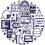# Ibraheem's divisibility theory

Ibraheem's divisibility theory is a new way for division with only addition and multiplication operations and only one easy division operation .
Where the known division ways uses a lot of division operations which take more time for computer to calculate than addition and multiplication .
The theory can help us to know if any number divides any prime number except 2,5 which are easy to check for them .

For example we can know if the number $m=1718364$ divide other prime number $p=13$ with only one division operation ,

If the prime number p units digit is $1$ :
We can write it as $x1$, where $x$ is the whole number without the units digit .
We have to multiply m units digit by $(9x+1 )$,and add it to (m without the units digit) ,
For example if $m=137$,and $p=11$
》 so $x=1$,
$m$ without the units digit = $13$,
$m$ units digit =$7$
So :p divides m $(11 divides 137)$ only if $11$ divides $7*(9*1+1)+13=83$ .

If p units digit is $3$ , we have to multiply the units digit of m by $3x+1$ and continue with the same way .

If p units digit is $7$ , we have to multiply the units digit of m by $7x+5$ and continue with the same way .

If p units digit is $9$ , we have to multiply the units digit of m by $x+1$ and continue with the same way .

We can repeat the same function until we reach small numbers , which we can divide by easily ,

Examples :

1)Does $31$ divide $1767$ ?
$31$ units digit is $1$
So we have to use $9x+1$ to multiply by ,
Where $x=3$, so we have to multiply by $9x+1=9*3+1=28$
$31$ divides $1767$ only if $31$ divides $176+7*28=372$
$31$ divides $1767$ only if $31$ divides $37+2*28=93$ .
$93/31=3$$31$ divides $93$》 31) divides $1767$ .

2)Does $43$ divide $58222$ ?
$43$ units digit is $3$
So we have to use $3x+1$ to multiply by ,
Where $x=4$, so we have to multiply by $3x+1=3*4+1=13$
$43$ divides $58222$ only if $43$ divides $5822+2*13=5848$ .
$43$ divides $58222$ only if $43$ divides $584+8*13=688$ .
$43$ divides $58222$ only if $43$ divides $68+8*13=172$
$43$ divides $58222$ only if \43) divides $(17+2*13=43$
$43/43=1$$43$ divides $43$$43$ divides $58222$ .

3)Does $7$ divide $3073$ ?
$7$ units digit is $7$
So we have to use $7x+5$ to multiply by ,
Where $x=0$, so we have to multiply by $7x+5=7*0+5=5$
$7$ divides $3073$ only if $7$ divides $307+3*5=322$ .
$7$ divides $3073$ only if $7$ divides $32+2*5=42$ .
$42/7=6$$7$ divides \42)》 $7$ divides $3073$ .

4)Does $379$ divide $136440$ ?
$379$ units digit is $9$
So we have to use $x+1$ to multiply by ,
Where $x=37$, so we have to multiply by $x+1=37+1=38$
$379$ divides $136440$ only if $379$ divides $13644+0*38=13644$ .
$379$ divides $136440$ only if $379$ divides $1364+4*38=1516$ .
$379$ divides $136440$ only if $379$ divides $151+6*38=379$ .
$379/379=1$$379$ divides $379$$379$ divides $136440$ .Note by ابراهيم فقرا
7 months, 3 weeks ago

This discussion board is a place to discuss our Daily Challenges and the math and science related to those challenges. Explanations are more than just a solution — they should explain the steps and thinking strategies that you used to obtain the solution. Comments should further the discussion of math and science.

When posting on Brilliant:

• Use the emojis to react to an explanation, whether you're congratulating a job well done , or just really confused .
• Ask specific questions about the challenge or the steps in somebody's explanation. Well-posed questions can add a lot to the discussion, but posting "I don't understand!" doesn't help anyone.
• Try to contribute something new to the discussion, whether it is an extension, generalization or other idea related to the challenge.

MarkdownAppears as
*italics* or _italics_ italics
**bold** or __bold__ bold
- bulleted- list
• bulleted
• list
1. numbered2. list
1. numbered
2. list
Note: you must add a full line of space before and after lists for them to show up correctly
paragraph 1paragraph 2

paragraph 1

paragraph 2

[example link](https://brilliant.org)example link
> This is a quote
This is a quote
    # I indented these lines
# 4 spaces, and now they show
# up as a code block.

print "hello world"
# I indented these lines
# 4 spaces, and now they show
# up as a code block.

print "hello world"
MathAppears as
Remember to wrap math in $$ ... $$ or $ ... $ to ensure proper formatting.
2 \times 3 $2 \times 3$
2^{34} $2^{34}$
a_{i-1} $a_{i-1}$
\frac{2}{3} $\frac{2}{3}$
\sqrt{2} $\sqrt{2}$
\sum_{i=1}^3 $\sum_{i=1}^3$
\sin \theta $\sin \theta$
\boxed{123} $\boxed{123}$

Sort by:

Great idea.

- 7 months, 3 weeks ago

Thank you 😄

- 7 months, 3 weeks ago